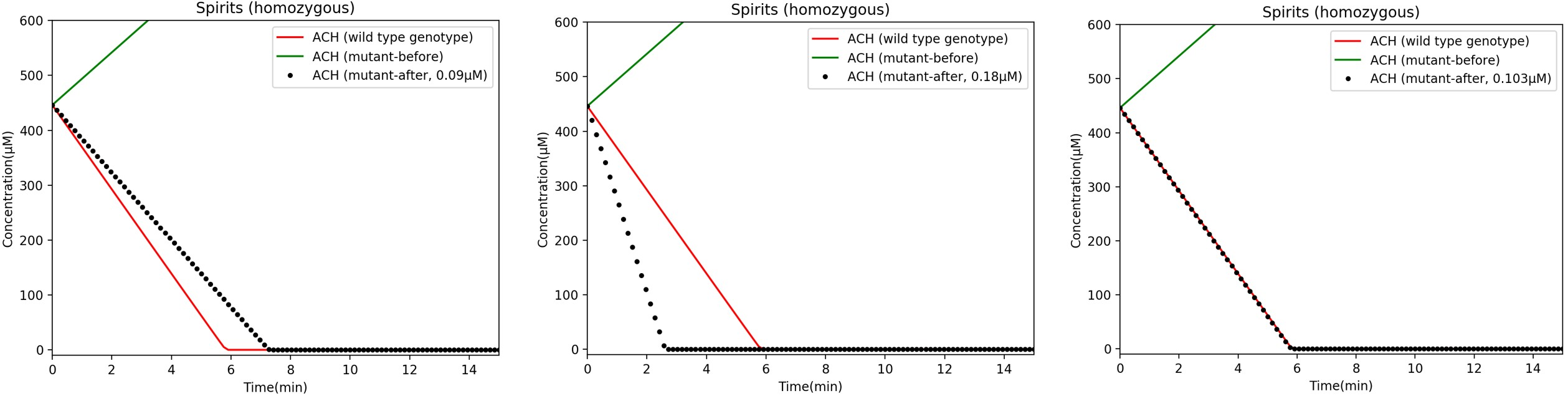TAS_Taipei

# MODELING

###### The two main objectives of our mathematical model are to:
1. Predict how much ALDH2 to consume in order to reduce acetaldehyde to match wild type ALDH2 levels.
2. Inform manufacturers how much ALDH2 needs to be put into the candies.

# OVERVIEW OF MODEL PARAMETERS

###### Our model treats the alcohol metabolism process as a series of steps mediated by two enzymes, alcohol dehydrogenase (ADH) and aldehyde dehydrogenase 2 (ALDH2). ADH converts ethanol to acetaldehyde (a toxic intermediate), and ALDH2 converts acetaldehyde to acetate (Figure 4-1).##### Figure 4-1: Alcohol Metabolism. Normally, ethanol is first converted to acetaldehyde (a toxic intermediate) by the enzyme alcohol dehydrogenase (ADH). Then, acetaldehyde is converted to acetate, which can be safely metabolized in the body, by the enzyme aldehyde dehydrogenase 2 (ALDH2). ALDH2*1 refers to wild type ALDH2. (Figure: Caroline C)
###### Since salivary acetaldehyde is the toxic intermediate we want to eliminate, we needed to first understand how fast acetaldehyde is made and metabolized in the mouth. In the ethanol metabolism pathway (Figure 4-1), four factors affect the acetaldehyde levels in the mouth after alcohol consumption.
1. Concentration of ethanol in the mouth
2. How fast ADH converts ethanol into acetaldehyde
3. Concentration of acetaldehyde in the mouth
4. How fast ALDH2*1 converts acetaldehyde into acetate

# FACTORS 1 & 3:Determining Ethanol & Acetaldehyde Concentrationsin the Mouth

##### Table 4-2. Salivary acetaldehyde levels after consuming one mouthful of different alcoholic beverages. Ethanol concentrations after 0.5 minutes were calculated as proportional to the reduction in acetaldehyde concentration after 0.5 minutes. (Lachenmeier et al., 2011)# FACTORS 2 & 4:Determining Enzyme Activities of ADH and ALDH2

###### We modeled both the ADH and ALDH2 reaction rates using Michaelis-Menten kinetics.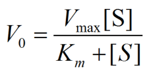# FACTOR 2:Determining Enzyme Activity of AlcoholDehydrogenase (ADH1B*2)

###### There are two main forms of alcohol dehydrogenase (ADH) involved in ethanol metabolism: ADH1B*1 (slower form) and ADH1B*2 (faster form). ALDH2 deficiency is an issue that mainly exists among East Asians, and we found that over 90% of East Asians have at least one ADH1B*2 allele (Eng et al., 2007). In our model, we made an assumption that all patients are heterozygous for ADH1B (*1/*2). From literature, we obtained the Km value for ADH1B*2 (which we assume to be the same for a slower form), and an effective rate of ethanol metabolism by ADH1B*1/*2 (0.82 uM/sec; unit conversion calculations are shown below; rate adapted from Yokoyama et al., 2016).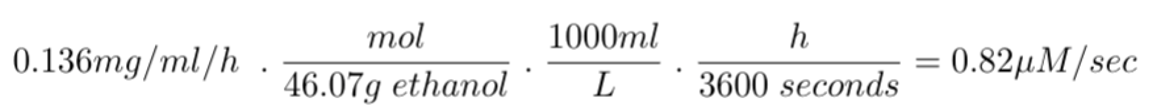###### We then created a Michaelis-Menten curve modeling the enzymes kinetics of the heterozygous ADH1B*1/*2 genotype.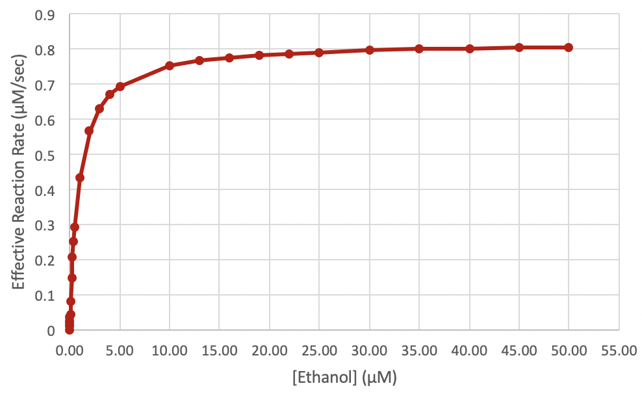# FACTOR 4:Determining Enzyme Activity of AldehydeDehydrogenase (ALDH2*1)

### Purified ALDH2*1 Activity at 37°C

###### In Prototype, we tested the enzymatic activity of purified ALDH2*1 at 37°C (Figure 3-2). In these experiments, we measured changes in NADH absorbance at 340 nm. To convert NADH absorbance into changes in concentration of NADH, we used the Beer-Lambert Law.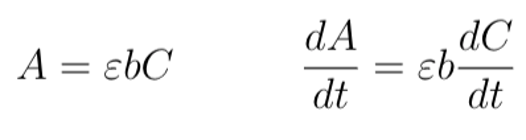##### Equation 2. Conversion of absorbance values to changes in NADH concentration. (Left) The variable A denotes NADH absorbance at 340 nm; the variable ε is a constant that denotes the NADH molar extinction factor at the same wavelength of light; the variable b is a constant that denotes the pathlength of the light; and C denotes the NADH concentration. (Right) The Beer-Lambert Law in the form of a differential equation, with changes in A relating to changes in C over time. We used this equation to determine the corresponding change in NADH concentration using a change in ∆ANADH from our functional test. (Figure: Justin L)
###### From the graph in Figure 3-2, we calculated the change in OD340 of NADH over a time period of 40 minutes. We first corrected the absorbance by subtracting the elution buffer control from the purified enzyme at t = 40 min. We then used the difference in absorbance from t = 0 min to t = 40 min to calculate dANADH/dt. By dividing dA/dt with the extinction coefficient εNADH(6220/M-1Cm-1, as indicated on the Megazyme kit manual) and the pathlength b (1 cm, which is the length of the cuvette), we calculated the rate of change in [NADH] over time (Figure 4-4). Because acetaldehyde, acetate, and NADH all react in a one-to-one ratio in the oxidation of acetaldehyde (Figure 4-1), a change in NADH concentration is equivalent to changes in acetaldehyde and acetate concentrations.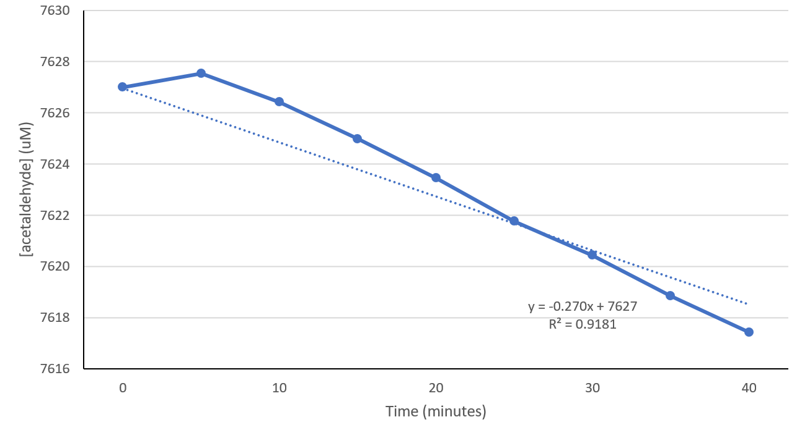##### Figure 4-4. Decrease in acetaldehyde concentration as a result of purified ALDH2*1 in artificial saliva at 37°C. The acetaldehyde concentrations were calculated from the raw absorbance values and the Beer-Lambert Law. The calculated acetaldehyde metabolism rate is 0.270 uM/min or 4.50 nM/sec. (Figure: Justin W)
###### Using a nanodrop spectrophotometer, the experimental concentration of purified ALDH2*1 was measured to be 0.222 nM. To determine if there was any enzyme degradation during the reaction, we used a rate equation based on the Michaelis-Menten model. The constant kcat represents the conversion rate of the ALDH2*1-acetaldehyde complex to acetate (1180/min; from Rashkovetsky et al., 1994). We used 4.50 nM/sec from the experiment above as the Vmax for ALDH2*1. We solved for [E0], the concentration of reacted ALDH2 enzymes, which turned out to be 0.229 nM. Because the measured and reacted ALDH2*1 concentrations have a 3% standard error, we concluded that enzyme degradation is negligible.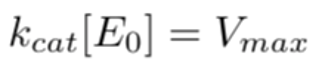### ALDH2*1-EcN Activity at 37°C

###### We next determined the rate of ALDH2*1-EcN activity at 37°C.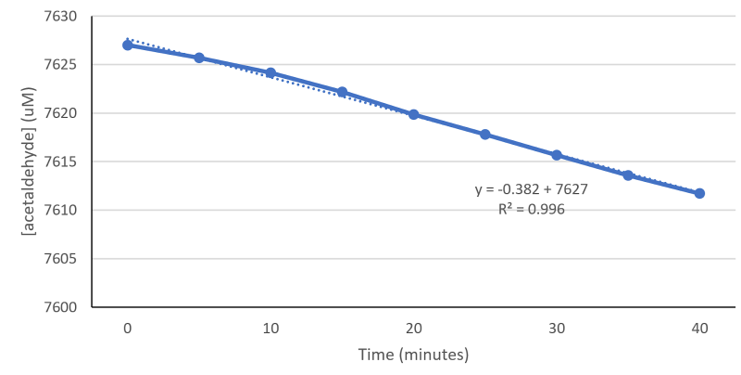##### Figure 4-5. Decrease in acetaldehyde concentration as a result of ALDH2*1 in 4.79*108 cells EcN at 37°C. Using experimental data from Figure 3-8 (see Prototype for details), absorbance values from ALDH2*2 in EcN were subtracted from ALDH2*1 in EcN. Following our assumption that bacteria cells contain other enzymes that may also convert NAD+ to NADH, this subtraction takes away the effects of other enzymes, and represents only the NADH converted by ALDH2*1. Absorbance values were then converted to acetaldehyde concentration, so we could determine the rate of acetaldehyde metabolism of the ALDH2*1 in our EcN probiotic. (Experiment & Figure: Justin W)
###### In our Prototype section, we documented functional tests for ALDH2*1-carrying EcN in artificial saliva at 37°C. Using experimental data from Figure 3-8 (in the Prototype section), absorbance values from ALDH2*2 in EcN were subtracted from ALDH2*1 in EcN. Because bacteria cells contain other enzymes that may also convert NAD+ to NADH, this subtraction allows us to determine the effects of other enzymes, and represents only the NADH converted by ALDH2*1. This correction was performed under the assumption that the enzymatic activity of ALDH2*2 was negligible. We then converted absorbance values to acetaldehyde concentration to determine the rate of acetaldehyde metabolism of the ALDH2*1 in our EcN bacteria. Here, we determined that our ALDH2*1-EcN bacteria converts acetaldehyde at a rate of 6.37 nM/sec at 37°C in artificial saliva. Knowing the enzyme activity of purified ALDH2*1 at 37°C (0.222 nM of purified ALDH2*1 converts 4.50 nM acetaldehyde/sec), and the rate of conversion in our ALDH2*1-EcN (6.37 nM acetaldehyde/sec), we set up a ratio to obtain the concentration of ALDH2*1, X, present in the EcN from our experiment (Figure 4-5).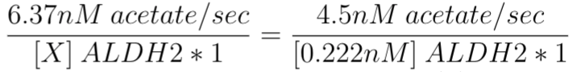# HOW MUCH ACETALDEHYDE SHOULD BEELIMINATED?

### Modeling Sequential Enzymatic Reactions##### Figure 4-6. A schematic diagram of a two-step sequential enzymatic reaction. In the metabolic pathway of ethanol to acetate, Substrate 1 represents ethanol; Enzyme 1 represents ADH1B*2; Substrate 2 represents acetaldehyde; Enzyme 2 represents ALDH2; Product represents acetate.
###### We constructed a system of two differential equations to model the change in concentrations of ethanol and acetaldehyde in a human’s mouth over time based on the pathway illustrated in Figure 4-6.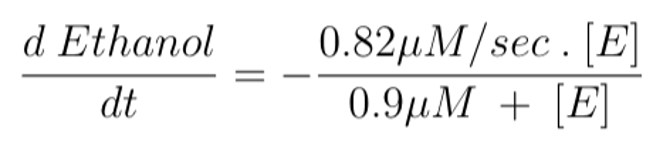##### Equation 4 models the change in ethanol concentration over time. We set it as equal to the enzymatic activity of ADH1B*2 enzymes (Figure 4-3). The symbol [E] represents ethanol concentration. This differential equation corresponds to the conversion rate of substrate 1 to substrate 2 in Figure 4-6.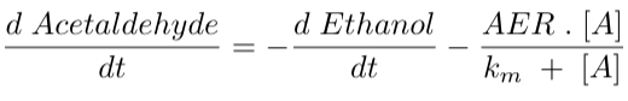### Calculating Required ALDH2 Levels

###### Through the program, we graphed acetaldehyde concentrations in the mouth over time after consuming different alcoholic beverages. There are three different ALDH2 genotypes (homozygous wild type ALDH2*1/*1; heterozygous ALDH2*1/*2; homozygous mutant ALDH2*2/*2), which process acetaldehyde at different rates (different Vmax values). Based on literature, we know that the heterozygous functions at 20-40% efficiency compared to homozygous wild type, while the homozygous mutant functions at 1-4% efficiency. The graphs below reflect the different efficiencies (20% and 1% were used to assume the worst case scenarios for the two mutant genotypes) (Gross et al., 2014; Chen et al., 2014).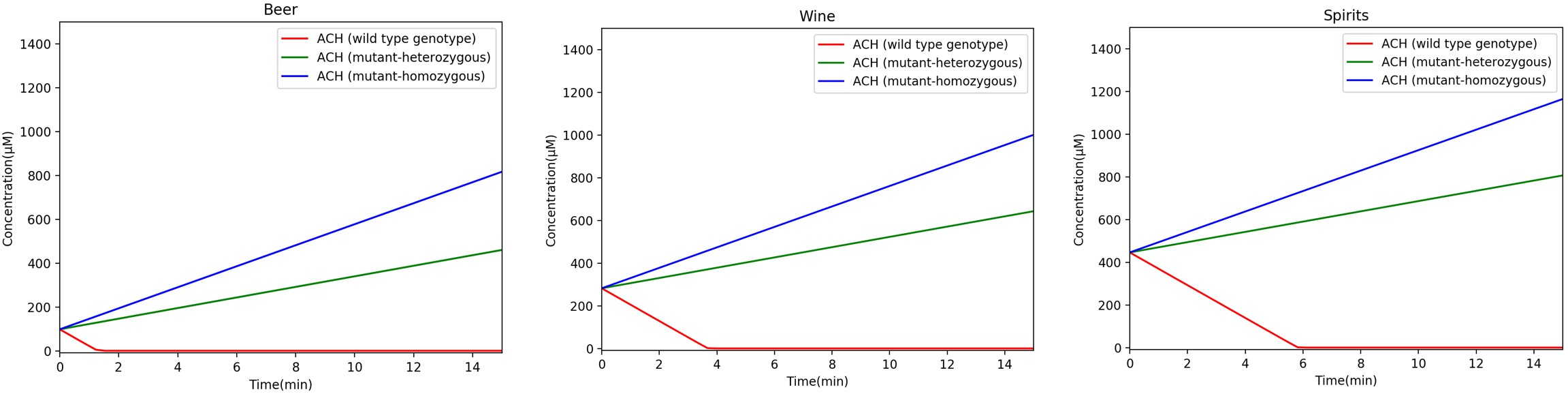##### Figure 4-7. Acetaldehyde concentration over time for three different types of alcohol (beer, wine, or spirits). The three curves in each graph represent acetaldehyde concentration over time for the different ALDH2 genotypes. Labels for each curve are provided in the legend.
###### As seen in Figure 4-7, homozygous wild-type ALDH2*1/*1 metabolizes acetaldehyde the fastest, while the homozygous mutant ALDH2*2/*2 metabolizes acetaldehyde the slowest. Our goal is to help homozygous mutant individuals metabolize acetaldehyde at the same rate as wild type individuals. We included the enzymatic activity for our purified ALDH2*1, which, when subtracted from Equation 5, should return acetaldehyde concentrations to wild type levels. This enzymatic activity can be adjusted by changing the enzyme concentration so that the acetaldehyde concentration matches levels found in wild type individuals.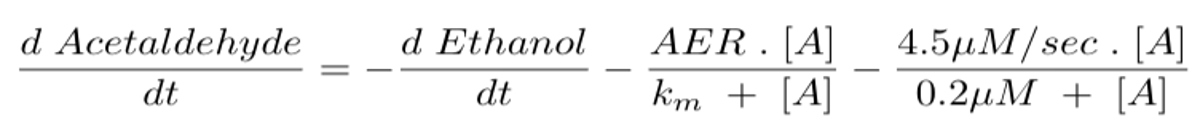##### Equation 6 models the rate of change in acetaldehyde concentration over time after additional ALDH2*1 enzymes are supplied to a homozygous mutant individual. The example enzymatic activity presented in the equation is the activity of 0.222 µM ALDH2*1 (from our purified enzyme functional test). The activity can be adjusted by adjusting the enzyme concentration.
###### Then, we plotted graphs to investigate the impact of different concentrations of additional ALDH2*1 on acetaldehyde concentrations for different genotypes (Figure 4-8).